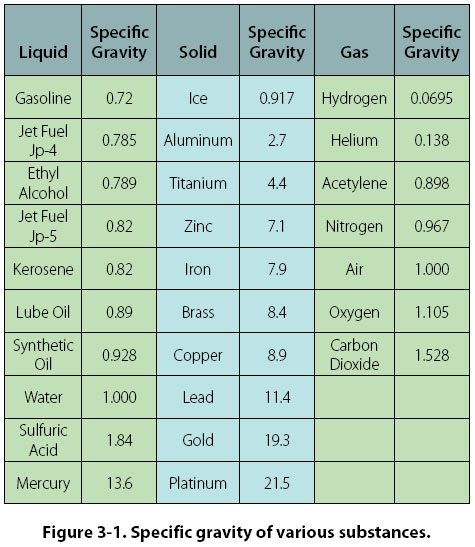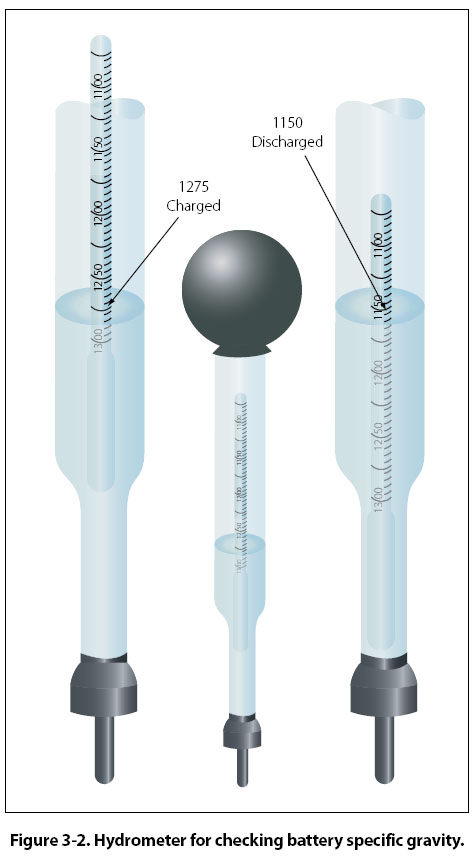Porosity Porosity means having pores or spaces where smaller particles may fit when a mixture takes place. This is sometimes referred to as granular — consisting or appearing to consist of small grains or granules. Impenetrability Impenetrability means that no two objects can occupy the same place at the same time. Thus, two portions of matter cannot at the same time occupy the same space. Density The density of a substance is its weight per unit volume. The unit volume selected for use in the English system of measurement is 1 cubic foot (ft3). In the metric system, it is 1 cubic centimeter (cm3). Therefore, density is expressed in pounds per cubic foot (lb/ft3) or in grams per cubic centimeter (g/cm3). To find the density of a substance, its weight and volume must be known. Its weight is then divided by its volume to find the weight per unit volume. For example, the liquid which fills a certain container weighs 1,497.6 lb. The container is 4 ft long, 3 ft wide, and 2 ft deep. Its volume is 24 ft3 (4 ft × 3 ft × 2 ft). If 24 ft3 of liquid weighs 1,497.6 lb, then 1 ft3 weighs 1,497.6 ÷ 24, or 62.4 lb. Therefore, the density of the liquid is 62.4 lb/ft3. This is the density of water at 4°C (Centigrade) and is usually used as the standard for comparing densities of other substances. In the metric system, the density of water is 1 g/cm3. The standard temperature of 4°C is used when measuring the density of liquids and solids. Changes in temperature will not change the weight of a substance, but will change the volume of the substance by expansion or contraction, thus changing its weight per unit volume. The procedure for finding density applies to all substances; however, it is necessary to consider the pressure when finding the density of gases. Pressure is more critical when measuring the density of gases than it is for other substances. The density of a gas increases in direct proportion to the pressure exerted on it. Standard conditions for the measurement of the densities of gases have been established at 0°C for temperature and a pressure of 76 cm of mercury (Hg). (This is the average pressure of the atmosphere at sea level.) Density is computed based on these conditions for all gases. Specific Gravity It is often necessary to compare the density of one substance with that of another. For this purpose, a standard is needed. Water is the standard that physicists have chosen to use when comparing the densities of all liquids and solids. For gases, air is most commonly used. However, hydrogen is sometimes used as a standard for gases. In physics, the word “specific" implies a ratio. Thus, specific gravity is calculated by comparing the weight of a definite volume of the given substance with the weight of an equal volume of water. The terms “specific weight" or “specific density" are sometimes used to express this ratio. The following formulas are used to find the specific gravity of liquids and solids. Specific Gravity = Weight of the substance/ Weight of an equal volume of water OR Specific Gravity = Density of the substance/ Density of water The same formulas are used to find the density of gases by substituting air or hydrogen for water. Specific gravity is not expressed in units, but as pure numbers. For example, if a certain hydraulic fluid has a specific gravity of 0.8, 1 ft3 of the liquid weighs 0.8 times as much as 1 ft3 of water: 62.4 times 0.8, or 49.92 lb. Specific gravity and density are independent of the size of the sample under consideration and depend only upon the substance of which it is made. See Figure 3-1 for typical values of specific gravity for various substances.A device called a hydrometer is used for measuring specific gravity of liquids. This device consists of a tubular glass float contained in a larger glass tube. [Figure 3-2] The larger glass tube provides the container for the liquid. A rubber suction bulb draws the liquid up into the container.There must be enough liquid to raise the float and prevent it from touching the bottom. The float is weighted and has a vertically graduated scale. To determine specific gravity, the scale is read at the surface of the liquid in which the float is immersed. An indication of 1000 is read when the float is immersed in pure water. When immersed in a liquid of greater density, the float rises, indicating a greater specific gravity. For liquids of lesser density the float sinks, indicating a lower specific gravity. An example of the use of the hydrometer is to determine the specific gravity of the electrolyte (batteryliquid) in an aircraft battery. When a battery is discharged, the calibrated float immersed in the electrolyte will indicate approximately 1150. The indication of a charged battery is between 1275 and 1310. The values 1150, 1275, and 1310 actually represent 1.150, 1.275, and 1.310. The electrolyte in a discharged battery is 1.15 times denser than water, and in a charged battery 1.275 to 1.31 times denser than water.
 ©AvStop Online Magazine                                                                                                                                                      Contact Us              Return To Books